Next: Exercises Up: Multi-Phase Systems Previous: Vapor Pressure

# Phase Transformations in Van der Waals Fluid

Consider a so-called van der Waals fluid whose equation of state takes the form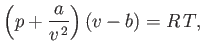(9.81)

where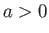,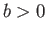are constants, and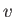is the molar volume. (See Sections 6.15 and 8.11.) It is helpful to write this equation of state in the reduced form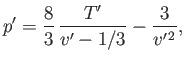(9.82)

where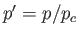,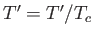, and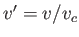. Here, the critical pressure, temperature and molar volume are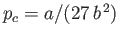,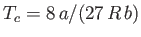, and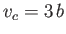, respectively. (See Section 6.15.)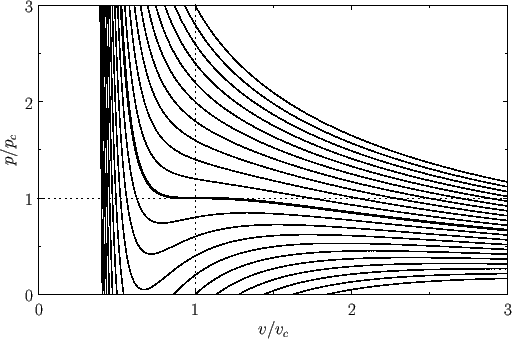Figure 9.3 shows the isotherms of the van der Waals equation of state plotted in the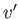-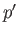plane. It can be seen that, for temperatures that exceed the critical temperature (i.e.,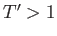), reducing the molar volume of the fluid causes its pressure to rise monotonically, eventually becoming infinite when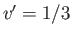(i.e., when the molecules are closely packed). On the other hand, for temperatures less than the critical temperature (i.e.,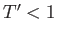), asis decreased the pressure rises, falls, and then rises again, which seems to imply that, for some molar volumes, compressing the fluid can cause its pressure to decrease. However, according to the analysis of Section 9.5, if a phase is such that compressing it causes its pressure to decrease (i.e., if its isothermal compressibility is negative) then the phase is unstable to density fluctuations. Thus, below the critical temperature, the stable states on a given isotherm are divided into two groups. The first group is characterized by relatively small molar volumes--these are liquid states. The second group is characterized by relatively large molar volumes--these are gas states. The liquid and gas states are separated by a set of unstable states. Above the critical temperature, the distinct liquid and gas states cease to exist--there is only a single stable fluid state. Consider the region of the-plane in which the states predicted by Equation (9.82) are unstable. It turns out that our analysis has broken down here because we assumed the existence of a single phase, whereas, in fact, in this region of the-plane, there exists a stable mixture of liquid and gas phases in equilibrium with one another. Let us investigate further.

At a given temperature and pressure, the true equilibrium state of a system is that which minimizes the Gibbs free energy. (See Section 9.4.) For the case of a van der Waals fluid,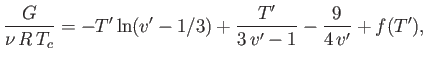(9.83)

where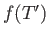is an unimportant function of the reduced temperature. (See Exercise 9.) In fact, defining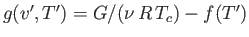, we can write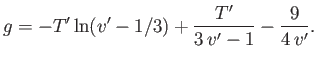(9.84)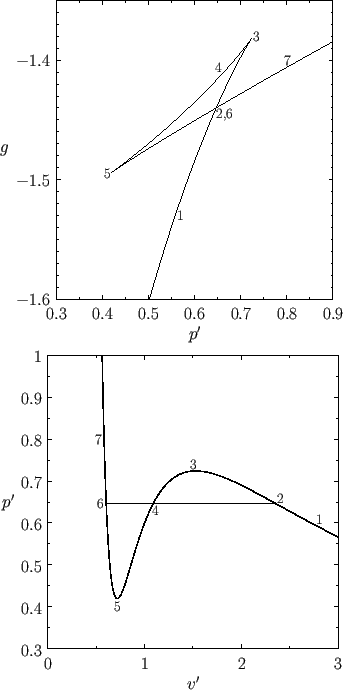Figure 9.4 shows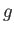plotted as a function offor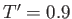. Also shown is the corresponding isotherm in the-plane. Although the van der Waals equation of state associates some pressures with more than one molar volume, the thermodynamically stable state is that with the lowest Gibbs free energy. Thus, the triangular loop in the graph ofversus(points 2-3-4-5-6) corresponds to unstable states. As the pressure is gradually increased, the system will go straight from point 2 to point 6, with an abrupt reduction in molar volume. Of course, this corresponds to a gas-liquid phase transition. At intermediate molar volumes, the thermodynamically stable state is a mixture of gaseous and liquid phases at the transition pressure, as illustrated by the straight-line in the-graph. The curved portion of the isotherm that is cut off by this straight-line correctly indicates what the allowed states would be if the fluid were homogeneous. However, these homogeneous states are unstable, because there is always another mixed state at the same pressure that possesses a lower Gibbs free energy.

The pressure at the phase transition can easily be determined from the graph ofversus. However, it is also possible to determine this pressure directly from the-isotherm. Note that the net change in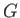in going around the triangular loop 2-3-4-5-6 in Figure 9.4 is zero. Hence,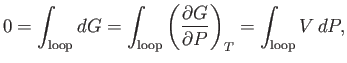(9.85)

where use has been made of Equation (6.116). It follows that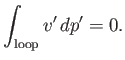(9.86)

In other words, the straight-line in the bottom panel of Figure 9.4 must be drawn in such a manner that the area enclosed between it and the curve 6-5-4 is equal to that enclosed between it and the curve 4-3-2. Drawing the straight-line so as to enclose equal areas in this manner is known as the Maxwell construction, after James Clerk Maxwell.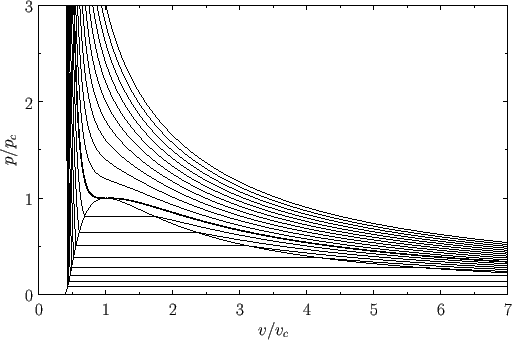Repeating the Maxwell construction for a variety of temperatures yields the phase diagram shown in Figure 9.5. [See J. Lekner, Am. J. Phys. 50, 161 (1982) for a practical method of implementing the Maxwell construction analytically. See, also, Exercise 7.] For each temperature, there is a well-defined pressure, known as the vapor pressure, at which the liquid-gas transformation takes place. Plotting this pressure versus the temperature yields the phase diagram shown in Figure 9.6. Note that the liquid-gas phase boundary disappears at the critical temperature (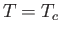), because there is no phase transformation above this temperature. The point at which the phase boundary disappears is called the critical point. The corresponding temperature, pressure, and volume are known as the critical temperature, pressure, and volume, respectively. At the critical point, the properties of the liquid and gas phases become identical.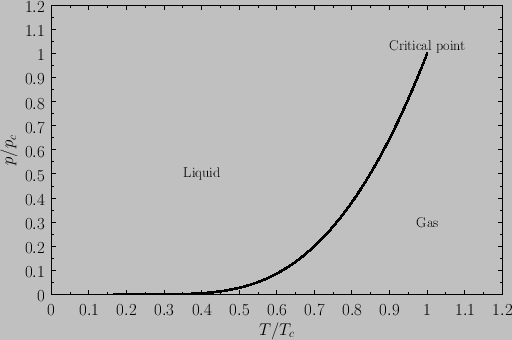Consider a phase transformation at some temperaturein which the gaseous phase has the molar volume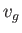, whereas the liquid phase has the molar volume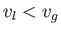. Now, the molar entropy of a van der Waals gas can be written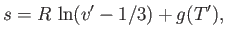(9.87)

where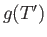is an unimportant function of the reduced temperature. (See Exercise 9.) Thus, the molar entropy increase associated with a liquid-gas phase transition is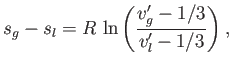(9.88)

where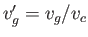and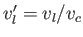. It follows from Equation (9.73) that the molar latent heat of vaporization of a van der Waals fluid takes the form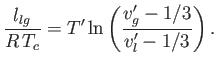(9.89)

Figure 9.7 shows the latent heat of vaporization of a van der Waals fluid plotted as a function of the temperature. It can be seen that the latent heat drops abruptly to zero as the temperature exceeds the critical temperature. Note the great similarity between this figure and Figure 7.10, which illustrates the variation of the spontaneous magnetization of a ferromagnet with temperature, according to mean-field theory. This similarity is not surprising, because it turns out that the simplifying assumptions that were made during the derivation of the van der Waals equation of state in Section 8.11 amount to a form of mean-field theory.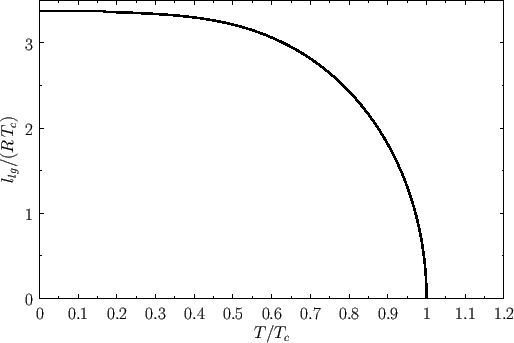Next: Exercises Up: Multi-Phase Systems Previous: Vapor Pressure
Richard Fitzpatrick 2016-01-25